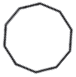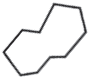# Decagon

A decagon is a polygon with 10 sides and 10 vertices. "Deca-" means "ten."

## Decagon classifications

Like other polygons, a decagon can be classified as regular or irregular.

Regular decagonIrregular dodecagonAll sides and interior angles are congruentSides and angles have various lengths

A decagon can also be classified as convex or concave.

A convex decagon is a polygon where no line segment between any two points on its boundary lies outside of it. None of the interior angles is greater than 180°. Think of a convex decagon as bulging outwards, like the regular decagon above.

Conversely, a concave decagon, like the irregular decagon shown above, has at least one-line segment that can be drawn between points on its boundary, but lies outside of it. Also, at least one of its interior angles is greater than 180°

A convex decagon does not have to be a regular decagon. Yet, a regular decagon is always a convex decagon.

The decagon above is convex but clearly has sides and angles that are not congruent. This decagon is irregular. Also, no part of any segment drawn between two boundary points, such as AB, lies outside of the decagon.

## Angles of a decagon

Decagons can be broken into a series of triangles by diagonals drawn from its vertices. This series of triangles can be used to find the sum of the interior angles of the decagon.

Diagonals are drawn from vertex A in the convex decagon below, forming 8 triangles. Similarly, 8 triangles can also be drawn in a concave decagon. Since the sum of the angles of a triangle is 180°, the sum of the interior angles of a dodecagon is 8 × 180° = 1440°.

A regular decagon has equal interior angle measures. Since 1440°/10 = 144°, each interior angle in a regular decagon has a measure of 144°. Also, each exterior angle has a measure of 36°.

### Did you know?

A regular decagon can be inscribed in a circle. Each vertex on the decagon lies on the circle. Also, the circle and decagon share the same center.